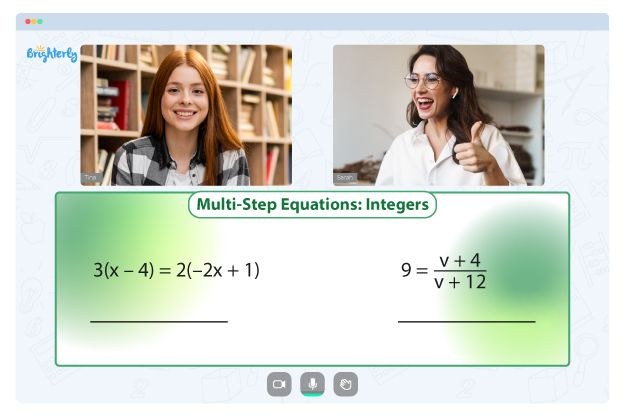# Solving Quadratic Equations By Factoring Worksheets

Factoring quadratic is a method of simplifying complex quadratic expressions to their simplest form, finding their roots, and arriving at an answer. The state of a quadratic polynomial is ax² + bx + c, where a, b, and c are fundamental values. It may be a complex task for kids to understand these concepts, especially when they are newly exposed to the topic. Let your kid try to solve quadratics by factoring worksheet, it is a practical resource to aid learning.

## Understanding the concept of quadratics

Solving quadratics entails finding a value(s) of a variable that satisfies a quadratic equation. Roots, solutions, or zeros are values that fulfill a quadratic equation. For instance, in the expression x²- 3x + 2 = 0, we can say x = 1 and x = 2 satisfy the equation. Therefore, the roots of the equation x² – 3x + 2 = 0 are x = 1 and x = 2. But if the values are not provided, you can solve the quadratic equations using the following methods:

• The process of factoring quadratic equations
• Applying the method of completing the square
• Graphing solutions to quadratic equations

Math for Kids

Is Your Child Struggling With Math?
1:1 Online Math TutoringThere are numerous questions for students to work on in the Brighterly’s quadratic equations worksheet. These worksheets differ depending on the school grade of your kids. However, their arrangement is similar – the approach to questions is stepwise, they range from the simplest problems to the toughest ones.

The tricky part of solving quadratics by factoring worksheet answers comprises questions with negative coefficients and squared terms greater than one. In the first question, the squared terms have a coefficient of one and all positive coefficients. As students overcome relatively easy questions, they proceed to the more complex ones. Also, children will encounter questions that require rearrangement before factoring.

Your kids’ ability to solve more straightforward questions at the beginning of the worksheets determines their readiness to deal with the complex ones. Also, these worksheets contain vital information that builds the foundation for further learning.### Solving Quadratic Equations By Factoring Worksheets PDF

Solving Quadratic Equations By Factoring Worksheet### Solving Quadratic Equations By Factoring Worksheets PDF### Solving Quadratic Equations By Factoring Worksheets PDF### Solving Quadratic Equations By Factoring Worksheets PDF

Solve Each Equation By Factoring Worksheet Answers

## Solve each equation by factoring worksheet answers in printable format

You can download the solving quadratics by factoring worksheet answers on the Brighterly’s website. With this resource, you don’t need to visit the site whenever you want your kids to study. All you have to do is download the PDF format and print the sheets. They contain answers, so your kids can learn while solving the problems.

### More Factoring Worksheets

Troubles with Equations?• Does your child need extra support with mastering of equations?
• Start lessons with an online tutor.

Is your child finding it hard to understand the concept of equations? An online tutor could be of help.